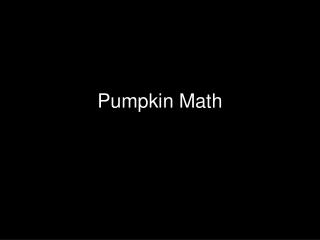DownloadDownload PresentationPumpkin Math

# Pumpkin Math

Télécharger la présentation## Pumpkin Math

- - - - - - - - - - - - - - - - - - - - - - - - - - - E N D - - - - - - - - - - - - - - - - - - - - - - - - - - -
##### Presentation Transcript

1. Pumpkin Math

2. Mathematical Investigations • Has multidimensional content • Is open-ended, with several acceptable solutions • Is an exploration requiring a pull period or longer to complete • Is centered on a theme or event • Is often embedded in a focus or driving question

3. Mathematical Investigations Involves process that include the following. • Researching outside sources • Collecting data • Collaborating with peers • Using multiple strategies to reach conclusions

4. NCTM Content Standards Involves the following content standards. • Algebra: represent, analyze, and generalize a variety of patterns with tables and graphs. • Data Analysis and Probability: formulate questions that can be addressed with data and to collect, organize, and display relevant data to answer them. • Measurement: appropriate use of measuring tools.

5. The Investigation Gabbard, G. (September, 2006) A Mathematical Cornucopia of Pumpkins. Teaching Children Mathematics, 68-78. Article provides objectives, needed materials, details of each day’s lesson. Investigation used in 3rd, 4th and 6th grades Four Day Investigation

6. Day One Brainstorm: What Measurements can we gather from the pumpkins?

7. Mass v. Weight What is the difference?

8. At this Point Five interesting Questions about Pumpkins.

9. Day Two • Volume of the Pumpkin • Cubing the Pumpkin

10. Volume • Archimedes • What can we do?

11. Cubing the Pumpkin • What is the smallest box that would hold your pumpkin?

12. Lining Up the Pumpkins Attributes are written on the pumpkin. Pumpkins are arranged in order according to an attribute (usually mass or volume). Descriptive statistics are determined.

13. Day Three Counting Seeds What method will you use? Relationships Find the following ratios EC to diameter PC to height EC to PC

14. Day Four Exploring with MS Excel Examining scatter plots EC to PC

15. Questions and Thoughts